15 November 2002

9 Basic Shapes

9.1 Introduction

SVG contains the following set of basic shape elements:

Mathematically, these shape elements are equivalent to a 'path' element that would construct the same shape. The basic shapes may be stroked, filled and used as clip paths. All of the properties available for 'path' elements also apply to the basic shapes.

9.2 The 'rect' element

The 'rect' element defines a rectangle which is axis-aligned with the current user coordinate system. Rounded rectangles can be achieved by setting appropriate values for attributes rx and ry.

 ``` ]]> ```

Attribute definitions:

x = "<coordinate>"
The x-axis coordinate of the side of the rectangle which has the smaller x-axis coordinate value in the current user coordinate system.
If the attribute is not specified, the effect is as if a value of "0" were specified.
Animatable: yes.
y = "<coordinate>"
The y-axis coordinate of the side of the rectangle which has the smaller y-axis coordinate value in the current user coordinate system.
If the attribute is not specified, the effect is as if a value of "0" were specified.
Animatable: yes.
width = "<length>"
The width of the rectangle.
A negative value is an error (see Error processing). A value of zero disables rendering of the element.
Animatable: yes.
height = "<length>"
The height of the rectangle.
A negative value is an error (see Error processing). A value of zero disables rendering of the element.
Animatable: yes.
rx = "<length>"
For rounded rectangles, the x-axis radius of the ellipse used to round off the corners of the rectangle.
A negative value is an error (see Error processing).
See the notes below about what happens if the attribute is not specified.
Animatable: yes.
ry = "<length>"
For rounded rectangles, the y-axis radius of the ellipse used to round off the corners of the rectangle.
A negative value is an error (see Error processing).
See the notes below about what happens if the attribute is not specified.
Animatable: yes.

If a properly specified value is provided for rx but not for ry, then the user agent processes the 'rect' element with the effective value for ry as equal to rx. If a properly specified value is provided for ry but not for rx, then the user agent processes the 'rect' element with the effective value for rx as equal to ry. If neither rx nor ry has a properly specified value, then the user agent processes the 'rect' element as if no rounding had been specified, resulting in square corners. If rx is greater than half of the width of the rectangle, then the user agent processes the 'rect' element with the effective value for rx as half of the width of the rectangle. If ry is greater than half of the height of the rectangle, then the user agent processes the 'rect' element with the effective value for ry as half of the height of the rectangle.

Mathematically, a 'rect' element can be mapped to an equivalent 'path' element as follows: (Note: all coordinate and length values are first converted into user space coordinates according to Units.)

• perform an absolute moveto operation to location (x+rx,y), where x is the value of the 'rect' element's x attribute converted to user space, rx is the effective value of the rx attribute converted to user space and y is the value of the y attribute converted to user space
• perform an absolute horizontal lineto operation to location (x+width-rx,y), where width is the 'rect' element's width attribute converted to user space
• perform an absolute elliptical arc operation to coordinate (x+width,y+ry), where the effective values for the rx and ry attributes on the 'rect' element converted to user space are used as the rx and ry attributes on the elliptical arc command, respectively, the x-axis-rotation is set to zero, the large-arc-flag is set to zero, and the sweep-flag is set to one
• perform a absolute vertical lineto to location (x+width,y+height-ry), where height is the 'rect' element's height attribute converted to user space
• perform an absolute elliptical arc operation to coordinate (x+width-rx,y+height)
• perform an absolute horizontal lineto to location (x+rx,y+height)
• perform an absolute elliptical arc operation to coordinate (x,y+height-ry)
• perform an absolute absolute vertical lineto to location (x,y+ry)
• perform an absolute elliptical arc operation to coordinate (x+rx,y)

Example rect01 shows a rectangle with sharp corners. The 'rect' element is filled with yellow and stroked with navy.

```<?xml version="1.0" standalone="no"?>
<!DOCTYPE svg PUBLIC "-//W3C//DTD SVG 1.1//EN"
"http://www.w3.org/Graphics/SVG/1.1/DTD/svg11.dtd">
<svg width="12cm" height="4cm" viewBox="0 0 1200 400"
xmlns="http://www.w3.org/2000/svg" version="1.1">
<desc>Example rect01 - rectangle with sharp corners</desc>
<!-- Show outline of canvas using 'rect' element -->
<rect x="1" y="1" width="1198" height="398"
fill="none" stroke="blue" stroke-width="2"/>
<rect x="400" y="100" width="400" height="200"
fill="yellow" stroke="navy" stroke-width="10"  />
</svg>
```Example rect02 shows two rounded rectangles. The rx specifies how to round the corners of the rectangles. Note that since no value has been specified for the ry attribute, it will be assigned the same value as the rx attribute.

```<?xml version="1.0" standalone="no"?>
<!DOCTYPE svg PUBLIC "-//W3C//DTD SVG 1.1//EN"
"http://www.w3.org/Graphics/SVG/1.1/DTD/svg11.dtd">
<svg width="12cm" height="4cm" viewBox="0 0 1200 400"
xmlns="http://www.w3.org/2000/svg" version="1.1">
<desc>Example rect02 - rounded rectangles</desc>
<!-- Show outline of canvas using 'rect' element -->
<rect x="1" y="1" width="1198" height="398"
fill="none" stroke="blue" stroke-width="2"/>
<rect x="100" y="100" width="400" height="200" rx="50"
fill="green" />
<g transform="translate(700 210) rotate(-30)">
<rect x="0" y="0" width="400" height="200" rx="50"
fill="none" stroke="purple" stroke-width="30" />
</g>
</svg>
```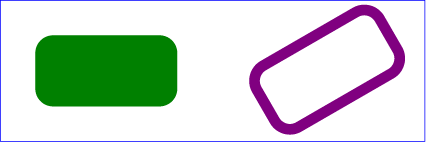9.3 The 'circle' element

The 'circle' element defines a circle based on a center point and a radius.

 ``` ]]> ```

Attribute definitions:

cx = "<coordinate>"
The x-axis coordinate of the center of the circle.
If the attribute is not specified, the effect is as if a value of "0" were specified.
Animatable: yes.
cy = "<coordinate>"
The y-axis coordinate of the center of the circle.
If the attribute is not specified, the effect is as if a value of "0" were specified.
Animatable: yes.
r = "<length>"
A negative value is an error (see Error processing). A value of zero disables rendering of the element.
Animatable: yes.

The arc of a 'circle' element begins at the "3 o'clock" point on the radius and progresses towards the "9 o'clock" point. The starting point and direction of the arc are affected by the user space transform in the same manner as the geometry of the element.

Example circle01 consists of a 'circle' element that is filled with red and stroked with blue.

```<?xml version="1.0" standalone="no"?>
<!DOCTYPE svg PUBLIC "-//W3C//DTD SVG 1.1//EN"
"http://www.w3.org/Graphics/SVG/1.1/DTD/svg11.dtd">
<svg width="12cm" height="4cm" viewBox="0 0 1200 400"
xmlns="http://www.w3.org/2000/svg" version="1.1">
<desc>Example circle01 - circle filled with red and stroked with blue</desc>
<!-- Show outline of canvas using 'rect' element -->
<rect x="1" y="1" width="1198" height="398"
fill="none" stroke="blue" stroke-width="2"/>
<circle cx="600" cy="200" r="100"
fill="red" stroke="blue" stroke-width="10"  />
</svg>
```9.4 The 'ellipse' element

The 'ellipse' element defines an ellipse which is axis-aligned with the current user coordinate system based on a center point and two radii.

 ``` ]]> ```

Attribute definitions:

cx = "<coordinate>"
The x-axis coordinate of the center of the ellipse.
If the attribute is not specified, the effect is as if a value of "0" were specified.
Animatable: yes.
cy = "<coordinate>"
The y-axis coordinate of the center of the ellipse.
If the attribute is not specified, the effect is as if a value of "0" were specified.
Animatable: yes.
rx = "<length>"
The x-axis radius of the ellipse.
A negative value is an error (see Error processing). A value of zero disables rendering of the element.
Animatable: yes.
ry = "<length>"
The y-axis radius of the ellipse.
A negative value is an error (see Error processing). A value of zero disables rendering of the element.
Animatable: yes.

The arc of an 'ellipse' element begins at the "3 o'clock" point on the radius and progresses towards the "9 o'clock" point. The starting point and direction of the arc are affected by the user space transform in the same manner as the geometry of the element.

Example ellipse01 below specifies the coordinates of the two ellipses in the user coordinate system established by the viewBox attribute on the 'svg' element and the transform attribute on the 'g' and 'ellipse' elements. Both ellipses use the default values of zero for the cx and cy attributes (the center of the ellipse). The second ellipse is rotated.

```<?xml version="1.0" standalone="no"?>
<!DOCTYPE svg PUBLIC "-//W3C//DTD SVG 1.1//EN"
"http://www.w3.org/Graphics/SVG/1.1/DTD/svg11.dtd">
<svg width="12cm" height="4cm" viewBox="0 0 1200 400"
xmlns="http://www.w3.org/2000/svg" version="1.1">
<desc>Example ellipse01 - examples of ellipses</desc>
<!-- Show outline of canvas using 'rect' element -->
<rect x="1" y="1" width="1198" height="398"
fill="none" stroke="blue" stroke-width="2" />
<g transform="translate(300 200)">
<ellipse rx="250" ry="100"
fill="red"  />
</g>
<ellipse transform="translate(900 200) rotate(-30)"
rx="250" ry="100"
fill="none" stroke="blue" stroke-width="20"  />
</svg>
```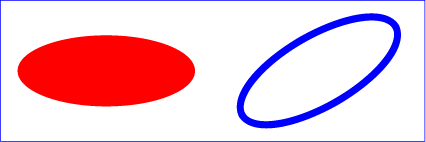9.5 The 'line' element

The 'line' element defines a line segment that starts at one point and ends at another.

 ``` ]]> ```

Attribute definitions:

x1 = "<coordinate>"
The x-axis coordinate of the start of the line.
If the attribute is not specified, the effect is as if a value of "0" were specified.
Animatable: yes.
y1 = "<coordinate>"
The y-axis coordinate of the start of the line.
If the attribute is not specified, the effect is as if a value of "0" were specified.
Animatable: yes.
x2 = "<coordinate>"
The x-axis coordinate of the end of the line.
If the attribute is not specified, the effect is as if a value of "0" were specified.
Animatable: yes.
y2 = "<coordinate>"
The y-axis coordinate of the end of the line.
If the attribute is not specified, the effect is as if a value of "0" were specified.
Animatable: yes.

Attributes defined elsewhere:

Mathematically, a 'line' element can be mapped to an equivalent 'path' element as follows: (Note: all coordinate and length values are first converted into user space coordinates according to Units.)

• perform an absolute moveto operation to absolute location (x1,y1), where x1 and y1 are the values of the 'line' element's x1 and y1 attributes converted to user space, respectively
• perform an absolute lineto operation to absolute location (x2,y2), where x2 and y2 are the values of the 'line' element's x2 and y2 attributes converted to user space, respectively

Because 'line' elements are single lines and thus are geometrically one-dimensional, they have no interior; thus, 'line' elements are never filled (see the 'fill' property).

Example line01 below specifies the coordinates of the five lines in the user coordinate system established by the viewBox attribute on the 'svg' element. The lines have different thicknesses.

```<?xml version="1.0" standalone="no"?>
<!DOCTYPE svg PUBLIC "-//W3C//DTD SVG 1.1//EN"
"http://www.w3.org/Graphics/SVG/1.1/DTD/svg11.dtd">
<svg width="12cm" height="4cm" viewBox="0 0 1200 400"
xmlns="http://www.w3.org/2000/svg" version="1.1">
<desc>Example line01 - lines expressed in user coordinates</desc>
<!-- Show outline of canvas using 'rect' element -->
<rect x="1" y="1" width="1198" height="398"
fill="none" stroke="blue" stroke-width="2" />
<g stroke="green" >
<line x1="100" y1="300" x2="300" y2="100"
stroke-width="5"  />
<line x1="300" y1="300" x2="500" y2="100"
stroke-width="10"  />
<line x1="500" y1="300" x2="700" y2="100"
stroke-width="15"  />
<line x1="700" y1="300" x2="900" y2="100"
stroke-width="20"  />
<line x1="900" y1="300" x2="1100" y2="100"
stroke-width="25"  />
</g>
</svg>
```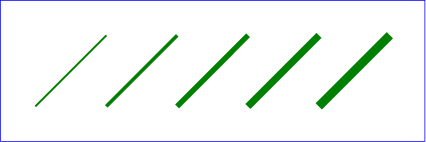9.6 The 'polyline' element

The 'polyline' element defines a set of connected straight line segments. Typically, 'polyline' elements define open shapes.

 ``` ]]> ```

Attribute definitions:

points = "<list-of-points>"
The points that make up the polyline. All coordinate values are in the user coordinate system.
Animatable: yes.

If an odd number of coordinates is provided, then the element is in error, with the same user agent behavior as occurs with an incorrectly specified 'path' element.

Mathematically, a 'polyline' element can be mapped to an equivalent 'path' element as follows:

• perform an absolute moveto operation to the first coordinate pair in the list of points
• for each subsequent coordinate pair, perform an absolute lineto operation to that coordinate pair.

Example polyline01 below specifies a polyline in the user coordinate system established by the viewBox attribute on the 'svg' element.

```<?xml version="1.0" standalone="no"?>
<!DOCTYPE svg PUBLIC "-//W3C//DTD SVG 1.1//EN"
"http://www.w3.org/Graphics/SVG/1.1/DTD/svg11.dtd">
<svg width="12cm" height="4cm" viewBox="0 0 1200 400"
xmlns="http://www.w3.org/2000/svg" version="1.1">
<desc>Example polyline01 - increasingly larger bars</desc>
<!-- Show outline of canvas using 'rect' element -->
<rect x="1" y="1" width="1198" height="398"
fill="none" stroke="blue" stroke-width="2" />
<polyline fill="none" stroke="blue" stroke-width="10"
points="50,375
150,375 150,325 250,325 250,375
350,375 350,250 450,250 450,375
550,375 550,175 650,175 650,375
750,375 750,100 850,100 850,375
950,375 950,25 1050,25 1050,375
1150,375" />
</svg>
```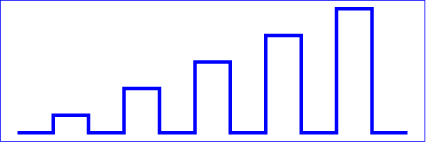9.7 The 'polygon' element

The 'polygon' element defines a closed shape consisting of a set of connected straight line segments.

 ``` ]]> ```

Attribute definitions:

points = "<list-of-points>"
The points that make up the polygon. All coordinate values are in the user coordinate system.
Animatable: yes.

If an odd number of coordinates is provided, then the element is in error, with the same user agent behavior as occurs with an incorrectly specified 'path' element.

Mathematically, a 'polygon' element can be mapped to an equivalent 'path' element as follows:

• perform an absolute moveto operation to the first coordinate pair in the list of points
• for each subsequent coordinate pair, perform an absolute lineto operation to that coordinate pair
• perform a closepath command

Example polygon01 below specifies two polygons (a star and a hexagon) in the user coordinate system established by the viewBox attribute on the 'svg' element.

```<?xml version="1.0" standalone="no"?>
<!DOCTYPE svg PUBLIC "-//W3C//DTD SVG 1.1//EN"
"http://www.w3.org/Graphics/SVG/1.1/DTD/svg11.dtd">
<svg width="12cm" height="4cm" viewBox="0 0 1200 400"
xmlns="http://www.w3.org/2000/svg" version="1.1">
<desc>Example polygon01 - star and hexagon</desc>
<!-- Show outline of canvas using 'rect' element -->
<rect x="1" y="1" width="1198" height="398"
fill="none" stroke="blue" stroke-width="2" />
<polygon fill="red" stroke="blue" stroke-width="10"
points="350,75  379,161 469,161 397,215
423,301 350,250 277,301 303,215
231,161 321,161" />
<polygon fill="lime" stroke="blue" stroke-width="10"
points="850,75  958,137.5 958,262.5
850,325 742,262.6 742,137.5" />
</svg>
```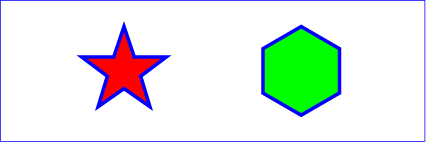9.8 The grammar for points specifications in 'polyline' and 'polygon' elements

The following is the Backus-Naur Form (BNF) for points specifications in 'polyline' and 'polygon' elements. The following notation is used:

• *: 0 or more
• +: 1 or more
• ?: 0 or 1
• (): grouping
• |: separates alternatives
• double quotes surround literals
```list-of-points:
wsp* coordinate-pairs? wsp*
coordinate-pairs:
coordinate-pair
| coordinate-pair comma-wsp coordinate-pairs
coordinate-pair:
coordinate comma-wsp coordinate
coordinate:
number
number:
sign? integer-constant
| sign? floating-point-constant
comma-wsp:
(wsp+ comma? wsp*) | (comma wsp*)
comma:
","
integer-constant:
digit-sequence
floating-point-constant:
fractional-constant exponent?
| digit-sequence exponent
fractional-constant:
digit-sequence? "." digit-sequence
| digit-sequence "."
exponent:
( "e" | "E" ) sign? digit-sequence
sign:
"+" | "-"
digit-sequence:
digit
| digit digit-sequence
digit:
"0" | "1" | "2" | "3" | "4" | "5" | "6" | "7" | "8" | "9"
wsp:
(#x20 | #x9 | #xD | #xA)+
```

9.9 Shape Module

Elements Attributes Content Model
path Core.attrib, Conditional.attrib, External.attrib, Style.attrib, transform, d, pathLength, GraphicalEvents.attrib, Paint.attrib, Paint.attrib, Opacity.attrib, Graphics.attrib, Cursor.attrib, Filter.attrib, Mask.attrib, GraphicalEvents.attrib, Clip.attrib, Marker.attrib (Description.class | Animation.class)*
rect Core.attrib, Conditional.attrib, Style.attrib, GraphicalEvents.attrib, Paint.attrib, Paint.attrib, Opacity.attrib, Graphics.attrib, Cursor.attrib, Filter.attrib, Mask.attrib, GraphicalEvents.attrib, Clip.attrib, x, y, width, height, rx, ry, transform (Description.class | Animation.class)*
circle Core.attrib, Conditional.attrib, Style.attrib, GraphicalEvents.attrib, Paint.attrib, Paint.attrib, Opacity.attrib, Graphics.attrib, Cursor.attrib, Filter.attrib, Mask.attrib, GraphicalEvents.attrib, Clip.attrib, cx, cy, r, transform (Description.class | Animation.class)*
line Core.attrib, Conditional.attrib, Style.attrib, GraphicalEvents.attrib, Paint.attrib, Paint.attrib, Opacity.attrib, Graphics.attrib, Cursor.attrib, Filter.attrib, Mask.attrib, GraphicalEvents.attrib, Clip.attrib, x1, y1, x2, y2, transform (Description.class | Animation.class)*
ellipse Core.attrib, Conditional.attrib, Style.attrib, GraphicalEvents.attrib, Paint.attrib, Paint.attrib, Opacity.attrib, Graphics.attrib, Cursor.attrib, Filter.attrib, Mask.attrib, GraphicalEvents.attrib, Clip.attrib, cx, cy, rx, ry, transform (Description.class | Animation.class)*
polyline Core.attrib, Conditional.attrib, Style.attrib, GraphicalEvents.attrib, Paint.attrib, Paint.attrib, Opacity.attrib, Graphics.attrib, Cursor.attrib, Filter.attrib, Mask.attrib, GraphicalEvents.attrib, Clip.attrib, points, transform (Description.class | Animation.class)*
polygon Core.attrib, Conditional.attrib, Style.attrib, GraphicalEvents.attrib, Paint.attrib, Paint.attrib, Opacity.attrib, Graphics.attrib, Cursor.attrib, Filter.attrib, Mask.attrib, GraphicalEvents.attrib, Clip.attrib, points, transform (Description.class | Animation.class)*

9.9.1 Shape Content Set

The Shape Module defines the Shape.class content set.

Content Set Name Elements in Content Set
Shape.class rect, circle, line, polyline, polygon, ellipse, path

9.10 DOM interfaces

The following interfaces are defined below: SVGRectElement, SVGCircleElement, SVGEllipseElement, SVGLineElement, SVGAnimatedPoints, SVGPolylineElement, SVGPolygonElement.

Interface SVGRectElement

The SVGRectElement interface corresponds to the 'rect' element.

IDL Definition
```interface SVGRectElement :
SVGElement,
SVGTests,
SVGLangSpace,
SVGExternalResourcesRequired,
SVGStylable,
SVGTransformable,
events::EventTarget {
};
```

Attributes
Corresponds to attribute x on the given 'rect' element.
Corresponds to attribute y on the given 'rect' element.
Corresponds to attribute width on the given 'rect' element.
Corresponds to attribute height on the given 'rect' element.
Corresponds to attribute rx on the given 'rect' element.
Corresponds to attribute ry on the given 'rect' element.

Interface SVGCircleElement

The SVGCircleElement interface corresponds to the 'circle' element.

IDL Definition
```interface SVGCircleElement :
SVGElement,
SVGTests,
SVGLangSpace,
SVGExternalResourcesRequired,
SVGStylable,
SVGTransformable,
events::EventTarget {
};
```

Attributes
Corresponds to attribute cx on the given 'circle' element.
Corresponds to attribute cy on the given 'circle' element.
Corresponds to attribute r on the given 'circle' element.

Interface SVGEllipseElement

The SVGEllipseElement interface corresponds to the 'ellipse' element.

IDL Definition
```interface SVGEllipseElement :
SVGElement,
SVGTests,
SVGLangSpace,
SVGExternalResourcesRequired,
SVGStylable,
SVGTransformable,
events::EventTarget {
};
```

Attributes
Corresponds to attribute cx on the given 'ellipse' element.
Corresponds to attribute cy on the given 'ellipse' element.
Corresponds to attribute rx on the given 'ellipse' element.
Corresponds to attribute ry on the given 'ellipse' element.

Interface SVGLineElement

The SVGLineElement interface corresponds to the 'line' element.

IDL Definition
```interface SVGLineElement :
SVGElement,
SVGTests,
SVGLangSpace,
SVGExternalResourcesRequired,
SVGStylable,
SVGTransformable,
events::EventTarget {
};
```

Attributes
Corresponds to attribute x1 on the given 'line' element.
Corresponds to attribute y1 on the given 'line' element.
Corresponds to attribute x2 on the given 'line' element.
Corresponds to attribute y2 on the given 'line' element.

Interface SVGAnimatedPoints

The SVGAnimatedPoints interface supports elements which have a 'points' attribute which holds a list of coordinate values and which support the ability to animate that attribute.

Additionally, the 'points' attribute on the original element accessed via the XML DOM (e.g., using the getAttribute() method call) will reflect any changes made to points.

IDL Definition
```interface SVGAnimatedPoints {
};
```

Attributes

Provides access to the base (i.e., static) contents of the points attribute.

Provides access to the current animated contents of the points attribute. If the given attribute or property is being animated, contains the current animated value of the attribute or property. If the given attribute or property is not currently being animated, contains the same value as 'points'.

Interface SVGPolylineElement

The SVGPolylineElement interface corresponds to the 'polyline' element.

IDL Definition
```interface SVGPolylineElement :
SVGElement,
SVGTests,
SVGLangSpace,
SVGExternalResourcesRequired,
SVGStylable,
SVGTransformable,
events::EventTarget,
SVGAnimatedPoints {};
```

Interface SVGPolygonElement

The SVGPolygonElement interface corresponds to the 'polygon' element.

IDL Definition
```interface SVGPolygonElement :
SVGElement,
SVGTests,
SVGLangSpace,
SVGExternalResourcesRequired,
SVGStylable,
SVGTransformable,
events::EventTarget,
SVGAnimatedPoints {};
```